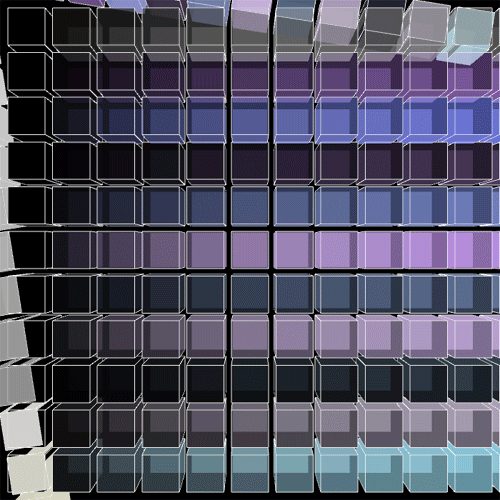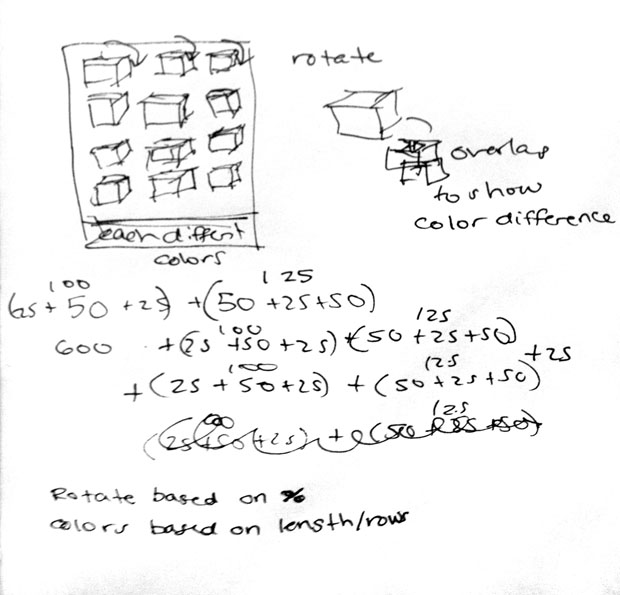# Amy Friedman

## 10 Feb 2015NEW LENTICULAR OBJECTOLD LENTICULAR OBJECTI was interested in utilizing color and transparency to overlap with one another to create new color variations. I wanted to have the boxes rotate downward one row at a time, but I had trouble figuring out how to fluidly rotate them the way I wanted. When exploring with overlapping objects, even when transparent they didnt blend the colors together as I had hoped, in some parts the sides of the cubes would change color but never the front faces. I tried moving the objects forwards and backwards in the Z direction to see if this would change the success of transparent blocks overlapping and nothing did the trick. I think the motions of the blocks could be improved as they are too fast. I appreciate that when the two block lines pass over each other they appear as though the are bending towards one another. I havent worked with variating color before it was interesting to experiment with.```//Amy Friedman copyright Feb 2015
//IACD coure by Golan Levin
//used sketch by Golan Levin for help

int nFramesInLoop = 10; // For lenticular export, REMEMBER TO CHANGE THIS to 10!
int nElapsedFrames;
boolean bRecording;
color colrand;
void setup(){
colrand =color(random(0,255), random(0,255),random(0,255),100);
size (700, 700, P3D);
bRecording = false;
nElapsedFrames = 0;
frameRate (nFramesInLoop);
//smooth();

}
//===================================================
void keyPressed() {
// Press a key to export frames to the output folder
bRecording = true;
nElapsedFrames = 0;
}

//===================================================
void draw() {

background(0);
// Compute a percentage (0...1) representing where we are in the loop.
float percentCompleteFraction = 0;
if (bRecording) {
percentCompleteFraction = (float) nElapsedFrames / (float)nFramesInLoop;
}
else {
float modFrame = (float) (frameCount % nFramesInLoop);
percentCompleteFraction = modFrame / (float)nFramesInLoop;
}

// Render the design, based on that percentage.
renderMyDesign (percentCompleteFraction);

// If we're recording the output, save the frame to a file.
if (bRecording) {
String myName = "amyfried";
saveFrame("output/"+ myName + "-loop-" + nf(nElapsedFrames, 4) + ".png");
nElapsedFrames++;
if (nElapsedFrames == nFramesInLoop) {
bRecording = false;
}
}
}

void renderMyDesign (float percent) {
float color_logs=percent;

float spacing=10;
float box_size=50;
float col, row;
fill(colrand, 40);
col=int(width/(box_size+spacing));
row=int(height/(box_size+spacing));

for (int i=0; i<=col; i++){
for (int j=0; j<=row-1; j++){
stroke(255);

float x_box = box_size+(box_size+spacing)*i;
float y_box = box_size+(box_size+spacing)*j;
float rotatingSquareAngle = percent * TWO_PI*.25;
float rotatingSquareAngle2 = percent * TWO_PI* -.25;

pushMatrix();
rotate (rotatingSquareAngle);
fill(255-30*i, 20);
translate(x_box, box_size);
//translate(x_box, y_box);
box(box_size);
popMatrix();

pushMatrix();
fill(colrand*j, 20);
rotate (rotatingSquareAngle2);
translate(box_size, y_box);
box(box_size);
popMatrix();

pushMatrix();
fill(colrand*j, 15*i);
translate(x_box, y_box);
box(box_size);
popMatrix();
}
}
}```